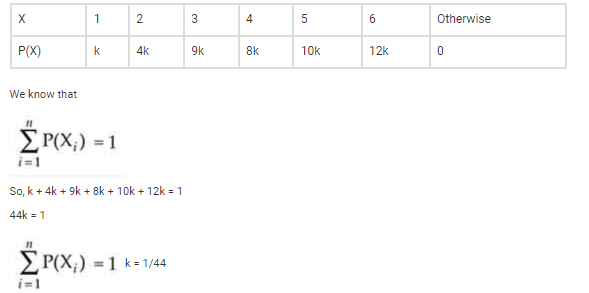# The probability distribution of a random variable x is given as under:Question:

The probability distribution of a random variable is given as under:

$\mathrm{P}(\mathrm{X}=x)=\left\{\begin{array}{l}k x^{2} \text { for } x=1,2,3 \\ 2 k x \text { for } x=4,5,6 \\ 0 \quad \text { otherwise }\end{array}\right.$

where is a constant. Calculate

(i) E(X)

(ii) E (3X2)

(iii) P(X ³ 4)

Solution:

The probability distribution of random variable X is given by:(i) E(X) =

= 1 x k + 2 x 4k + 3 x 9k + 4 x 8k + 5 x 10k + 6 x 12k

= k + 8k + 27k + 32k + 50k + 72k = 190k

= 190 x 1/44 = 95/22 = 4.32 (approx.)

(ii) E(X2) = 3(k + 4 x 4k + 9 x 9k + 16 x 8k + 25 x 10k + 36 x 12k)

= 3(k + 16k + 81k + 128k + 250k + 432k) = 3(908k)

= 3 x 908 x 1/44 = 2724/44 = 61.9 (approx.)

(iii) P(X ≥ 4) = P(X = 4) + P(X = 5) + P(X = 6)

= 8k + 10k + 12k = 30k

= 30 x 1/44 = 15/22